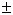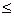edHelper subscribers - Create a new printable

 Name _____________________________Date ___________________
Solve each equation.
1.
 6x2 - 864 = 0

 2 -3x2 + 300 = 0
3.
-8
 25
x2 +
58
 45
=
8
 25

Solve each equation by factoring.
4.
1
 12
x2 + 120  =  -27x

5.
 -6x2 - 14x + 33 = -7x2

6.
-2
 13
x2 +
 13
=
-2
 12
x2 +
 12
x

Solve each equation by completing the square.
7.
 -3x2 - 36x - 21 = 0

 8 5x2 - 9x + 4 = 0
9.
-3
 13
x2 +
1
 23
x  =
1
 13
x -
 23

Use the discriminant to determine the nature of each quadratic equation's roots.
 10 9x2 + 12x + 4 = 0
11.
4
 12
x2 + 6x + 2  =  0

12.
8
 13
x2 + 50x -
8
 13
=  25

Use the quadratic formula to solve each equation.
13.
24
 34
x2 -
82
 12
x  =  -33

 14 -5x2 + 12x - 7 = 0
15.
 -36x + 16 = -20x2

Use the quadratic formula to solve each equation.
16.
 -10x2 + 6x - 2 = -5x2

17.
27
 12
x2 + 10x +
7
 12
=  0

18.
 -5x + 8 = -10x2 + 6

Write a quadratic equation that has the given roots.
19.
 { 2 , -5 }

20.
 { 4 , 2 }

21.
 -22i

Find the vertex and axis of symmetry of the parabola.
 22 -12x2 - 5x + 12 = 0
 23 -4x2 + 2x + 11 = 0
 24 3x2 + 7x + 2 = 0

Find the vertex and axis of symmetry of the parabola.
25.
 y = -x2 - 5x + 12

26.
 y = 36x2 - 12x - 8

27.
y  =  3x2 - 6x -
 34

Write an equation for a parabola that passes through the given points.
 28 (6, 27), (5, 18), (-4, 27), (-3, 18)

Graph each equation.
 29 y = x2 - 10x + 19
 30 y = -x2 + 2x + 2

Solve each inequality.
 31 x2 + 3x + 20
 32 -8x2 - 10x + 3  <  0
 33 5x2 - x - 4  >  0

Sample
This is only a sample worksheet.

edHelper subscribers - Create a new printable